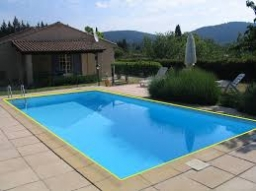# Water-wetted 35193

The block-shaped pool with dimensions of 25 m and 12.5 m and a depth of 2 m is filled with 4/5 of water. Calculate the area of water-wetted areas.

S =  432.5 m2

### Step-by-step explanation:Did you find an error or inaccuracy? Feel free to write us. Thank you!

Tips for related online calculators
Need help calculating sum, simplifying, or multiplying fractions? Try our fraction calculator.

#### Grade of the word problem:

We encourage you to watch this tutorial video on this math problem: